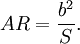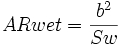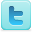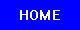﻿ Aspect Ratio Experiments and Background Information
Aspect Ratio
Experiments and Background Information

 Experiments Aspect Ratio Calculator [View Experiment] Mechanisms underlying the perceived angular velocity of a rigidly rotating object [View Experiment] Recent progress of low-aspect ratio experiments [View Experiment] Further Technion Studies on Aeroelastic Experiments of Composite High-Aspect-Ratio Wing Model [View Experiment] Aspect Ratio Definition In aerodynamics, the aspect ratio is an airplane's wing's span divided by its standard mean chord (SMC). Basics The aspect ratio can be calculated more easily as span squared divided by wing area:$AR = {b^2 \over S}.$ Informally, a high aspect ratio indicates long, narrow wings, whereas a low aspect ratio indicates short, stubby wings. Aspect ratio and planform are powerful indicators of the general performance of a wing, although the aspect ratio as such is only a secondary indicator. The wingspan is the crucial component of the performance. This is because an airplane derives its lift from a roughly cylindrical tube of air that is affected by the craft as it moves, and the diameter of that cylindrical tube is equal to the wingspan. Thus a large wingspan is working on a large cylinder of air, and a small wingspan is working on a small cylinder of air. The smaller cylinder of air must be pushed downward by a greater amount in order to produce an equal upward force; the aft-leaning component of this change in velocity is proportional to the induced drag. Therefore a large downward velocity is proportional to a large induced drag. The interaction between undisturbed air outside the cylindrical tube of air, and the downward-moving cylindrical tube of air occurs at the wing's tips, and can be seen as wingtip vortices. There are several reasons why all aircraft do not have high aspect wings: Structural: A long wing has higher bending stress for a given load than a short one, which requires stronger structure to withstand. Also, longer wings have greater deflection for a given load, and in some applications this deflection is undesirable (e.g. if the deflected wing interferes with aileron movement). Parasite Drag: While high aspect wings create less induced drag, they have greater parasite drag, (drag due to shape, frontal area, and friction). This is because, for an equal wing area, the average chord (length in the direction of wind travel over the wing) is smaller. Due to the effects of Reynolds Number, the value of the section-drag coefficient is an inverse logarithmic function of the characteristic length of the surface, which means that, even if two wings of the same area are flying at equal speeds and using equal angles of attack, the section drag coefficient is slightly higher on the wing with the smaller chord. However, this variation is very small when compared to the variation in induced drag with changing wingspan (for example, Airplane Aerodynamics by Dommasch/Sherby/Connolly, published by Pitman Publishing in 1961, calculated on page 128 the ratio of a specific airfoil contour (NACA 23012) at typical lift coefficients: Section Drag Ratio = (chord ratio)^0.129, which means that a 20 percent increase in chord length would decrease the section drag coefficient by 2.4 percent) Practicality: low aspect ratios have a greater useful internal volume, since the maximum thickness is greater, which can be used to house the fuel tanks, retractable landing gear and other systems. Variable Aspect Ratio: Aircraft which approach or exceed the speed of sound sometimes incorporate variable aspect-ratio wing structures. This is due to the difference in fluid behavior in the subsonic and transonic/supersonic regimes. In subsonic flow, the most significant component of drag is the induced drag, which is a function of the circular tube of air affected by the wing's passage. However, as the flow becomes supersonic, the shock wave first generated along the wing's upper surface causes a huge drag on the aircraft, and this drag is proportional to the length of the wing - the longer the wing, the longer the shock wave. Thus a long wing, valuable at low speeds, becomes a detriment at transonic speeds. If the aircraft can stand the extra weight and complexity of a moveable wing, the swing-wing provides a solution to this problem. Aspect ratio of bird wings: High aspect ratio wings abound in nature. Most birds which have to cover long distances e.g. on migratory routes have a high aspect ratio, and with tapered or elliptical wingtips. This is particularly noticeable on soaring birds such as albatrosses and eagles. By contrast, hawks of the genus Accipiter such as the Eurasian Sparrowhawk have low aspect ratio wings (along with long tails) for maneuverability. The wetted aspect ratio is a good indication of the aerodynamic efficiency of an aircraft. It is a better measure than the aspect ratio. It is defined as:$ARwet = {b^2 \over Sw}$ where Sw is the wetted surface of the whole aircraft in contrast to the wing area used for the definition of the aspect ratio. A good example of this is the Boeing B-47 and Avro Vulcan. Both aircraft have very similar performance although they are radically different. The B-47 has a high aspect ratio wing, while the Avro Vulcan is a low aspect ratio blended wing body. They have, however, a very similar wetted aspect ratio. Source: Wikipedia (All text is available under the terms of the GNU Free Documentation License and Creative Commons Attribution-ShareAlike License.) Useful Links Science Fair Projects Resources Engineering Science Fair Books